• matlab天线方向图计算-天线方向图程序.rar matlab天线方向图计算matlab
• 由已知的方向图函数画出天线方向图，得到三维和二维图形
• 方向图上面不能得到天线增益，由方向图得到的是方向系数。天线增益=方向系数 * 天线效率。所以 方向系数 大于 增益 是肯定的。
• 天线方向图绘制程序包，根据数据绘制三维方向图，还有阵列天线合成程序包
• 方向图上面不能得到天线增益，由方向图得到的是方向系数。天线增益=方向系数 * 天线效率。所以 方向系数 大于 增益 是肯定的。
• 天线方向图matlab代码天线模式可视化脚本 工程终端教程中的天线方向图绘制代码。 代码以 MATLAB、Python 和 Julia 编程语言提供。
• 天线方向图的形成原理 用MATLAB画出2根天线方向图 代码如下： f=3e10; lamda=(3e8)/f; beta=2.*pi/lamda; n=2; t=0:0.01:2*pi; d=lamda/4; W=beta.*d.*cos(t); z1=((n/2).*W)-n/2*...


天线方向图的形成原理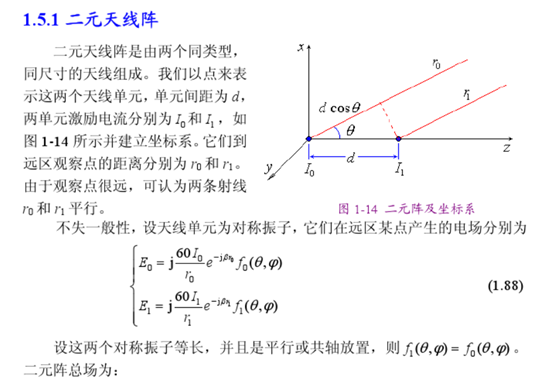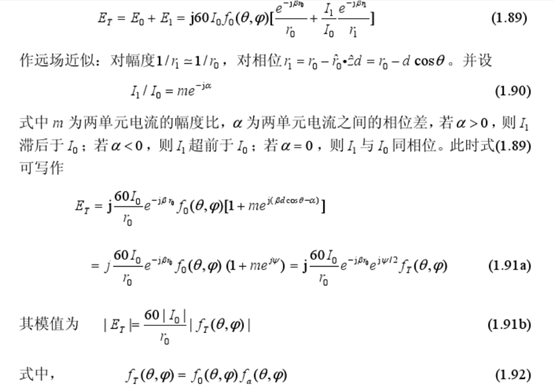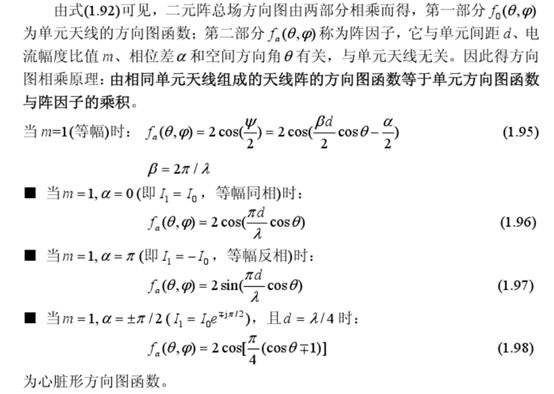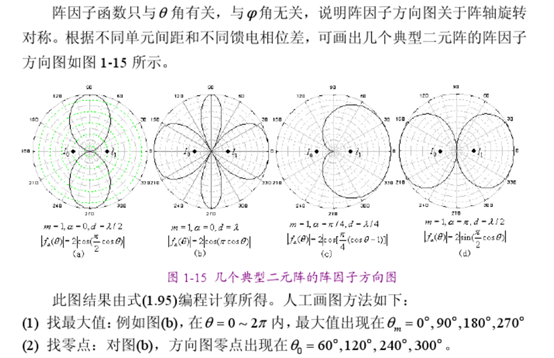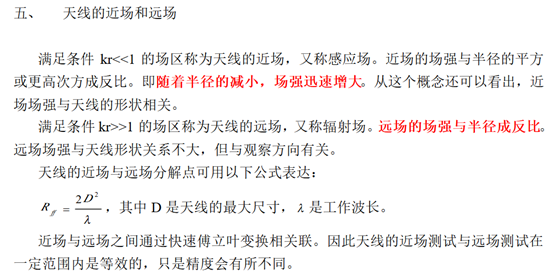用MATLAB画出2根天线方向图

代码如下：

 f=3e10;
lamda=(3e8)/f;
beta=2.*pi/lamda;
n=2;
t=0:0.01:2*pi;
d=lamda/4;
W=beta.*d.*cos(t);
z1=((n/2).*W)-n/2*beta*d;
z2=((1/2).*W)-1/2*beta*d;
F1=sin(z1)./(n.*sin(z2));
K1=abs(F1);
polar(t,K1);


MATLAB结果：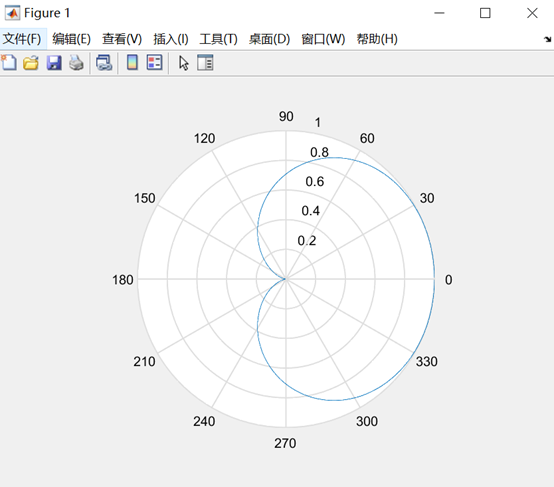假设阵元间距为波长的四分之一，天线元数目是10个

代码如下：

f=3e10;
lamda=(3e8)/f;
beta=2.*pi/lamda;     //β=2π/λ 波数
n=10;                 //天线阵元数目
t=0:0.01:2*pi;
d=lamda/4;           //假设阵元间距为波长的四分之一
W=beta.*d.*cos(t);
z1=((n/2).*W)-n/2*beta*d;
z2=((1/2).*W)-1/2*beta*d;
F1=sin(z1)./(n.*sin(z2));
K1=abs(F1);         //函数对数组元素进行绝对值处理的函数
polar(t,K1);


MATLAB结果：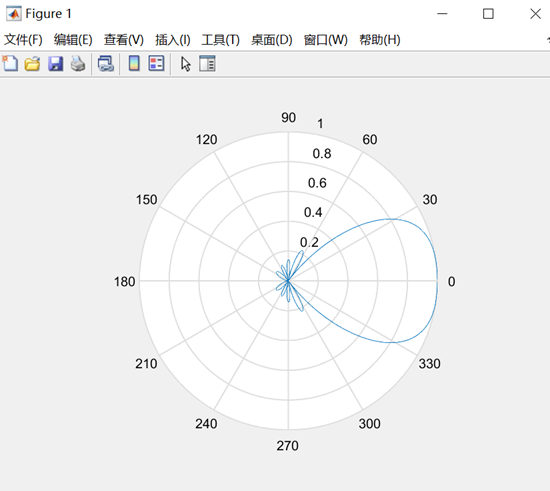展开全文• 利用LMS算法和SMI算法作的阵列天线方向图，干扰信号可选幅度调制方式或者角度调制。
• 在已有的理论基础上，考虑Stokes参数之间的互相影响，提出了一种高效、简便的全极化微波辐射计天线方向图校正算法，同时对该算法进行了较为深入的误差分析，仿真结果表明，该算法对天线方向图交叉极化校正效果明显。
• 　天线是雷达的重要组成部分，天线方向图的测试在雷达性能测试中占有极其重要的位置。早期人们采用手动法进行方向图测量，数据的录取、方向图的绘制以及参数的计算都是手工方式，操作复杂，工作量大，耗时长，精度低...
• 构建直线阵列、圆阵列和平面阵列天线的数学模型并推导其阵因子表达式,借助Matlab对3种不同类型的阵列天线方向图进行仿真研究。对比阵元数、波长、阵元间距等参数对不同类型阵列天线方向图的影响,仿真结果表明:直线阵...
• 讲解了MATLAB画偶极子天线方向图的步骤！ 方向性函数F（θ，Φ）是表征辐射场在不同方向辐射特性的重要关系式，对它的分析和认识如果仅仅停留在方向性函数以及公式中各参数的讨论上，很难理解天线辐射场的空间分布...
• 阵列天线方向图-均匀直线matlab仿真 设一直线阵列由20个阵元按半倍波长等间隔排列组成，所有阵元等幅全向，波长为1m，即阵列孔径为9.5m，天线波束指向为0°。
• ## 基于matlab的天线方向图

万次阅读 多人点赞 2019-03-17 18:59:39
影响天线方向图的因素 方向图随N变化的代码 sita=-pi/2:0.01:pi/2 lamda=0.03 d=lamda/4n1=4 beta=2*pi*d*sin(sita)/lamda z11=(n1/2)*beta z21=(1/2)*beta f1=sin(z11)./(n1*sin(z21)) F1=abs(f1) figure...
影响天线方向图的因素
方向图随N变化的代码
sita=-pi/2:0.01:pi/2
lamda=0.03
d=lamda/4n1=4
beta=2*pi*d*sin(sita)/lamda
z11=(n1/2)*beta
z21=(1/2)*beta
f1=sin(z11)./(n1*sin(z21))
F1=abs(f1)
figure(1)
plot(sita,F1,'b')
hold on
n2=6
beta=2*pi*d*sin(sita)/lamda
z12=(n2/2)*beta
z22=(1/2)*beta
f2=sin(z12)./(n1*sin(z22))
F2=abs(f2)
plot(sita,F2,'r')
hold on
n3=9
beta=2*pi*d*sin(sita)/lamda
z13=(n3/2)*beta
z23=(1/2)*beta
f3=sin(z13)./(n1*sin(z23))
F3=abs(f3)plot(sita,F3,'k')
hold off
grid on
ylabel('amplitude')title('方向图与阵列个数的关系')legend('n=4’','n=6','n=9')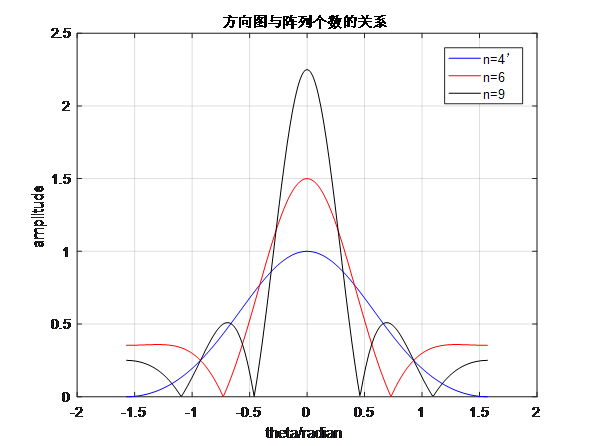结论：随着阵列个数的n的增加，方向图衰减的越快，效果就越好

方向图随波长lamda变化的代码
sita=-pi/2:0.01:pi/2
n=9
d=0.0002
lamda1=0.002
beta=2*pi*d*sin(sita)/lamda1
z11=(n/2)*beta
z21=(1/2)*beta
f1=sin(z11)./(n1*sin(z21))
F1=abs(f1)
figure(1)plot(sita,F1,'b')
hold on
lamda2=0.003
beta=2*pi*d*sin(sita)/lamda2
z12=(n/2)*betaz22=(1/2)*beta
f2=sin(z12)./(n1*sin(z22))
F2=abs(f2)plot(sita,F2,'r')
hold on
lamda3=0.004
beta=2*pi*d*sin(sita)/lamda3
z13=(n/2)*beta
z23=(1/2)*beta
f3=sin(z13)./(n1*sin(z23))
F3=abs(f3)plot(sita,F3,'k')
hold off
grid on
ylabel('amplitude')title('方向图与波长的关系')
legend('lamda1=0.002','lamda2=0.003','lamda3=0.004')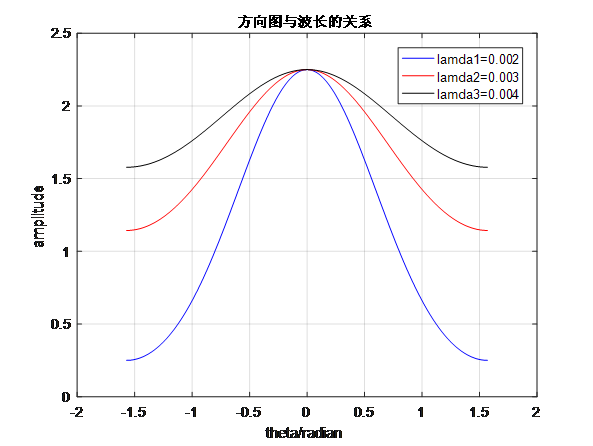结论：随着波长lamda的增大，方向图衰减的越慢，收敛性越不是很好

方向图随阵元间的距离变化的代码
 sita=-pi/2:0.01:pi/2
n=9
lamda=0.3
d1=0.01
beta=2*pi*d*sin(sita)/lamda1
z11=(n/2)*beta
z21=(1/2)*beta
f1=sin(z11)./(n1*sin(z21))
F1=abs(f1)
figure(1)
plot(sita,F1,'b')
hold on
d2=0.0075
beta=2*pi*d*sin(sita)/lamda2
z12=(n/2)*beta
z22=(1/2)*betaf2=sin(z12)./(n1*sin(z22))
F2=abs(f2)
plot(sita,F2,'r')
hold on
d3=0.005
beta=2*pi*d*sin(sita)/lamda3
z13=(n/2)*betaz23=(1/2)*beta
f3=sin(z13)./(n1*sin(z23))
F3=abs(f3)
plot(sita,F3,'k')
hold off
grid on
ylabel('amplitude')title('方向图与d的关系')
legend('d1=0.01','d2=0.0075','d3=0.005')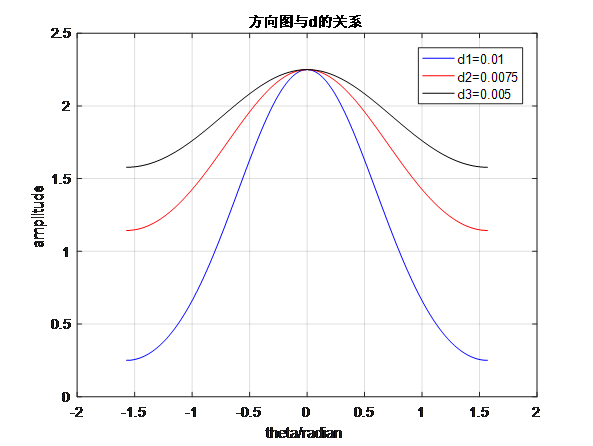结论：随着阵元之间间隔的增加，方向图衰减越快，主次瓣的差距越大，次瓣衰减越快，效果越好。

三维
a=linspace(0,2*pi)
b=linspace(0,pi)
f=sin((cos(a).*sin(b)-1)*(14/2)*pi)./(sin((cos(a).*sin(b)-1)*pi/2)*14)
polar(a,f.*sin(b))
title('14元端射式H面，d=/2,相位=滞后')
y1=(f.*sin(a))'*cos(b)
z1=(f.*sin(a))'*sin(b)
x1=(f.*cos(a))'*ones(size(b))
surf(x1,y1,z1)
axis equal title('14元端射式三维图')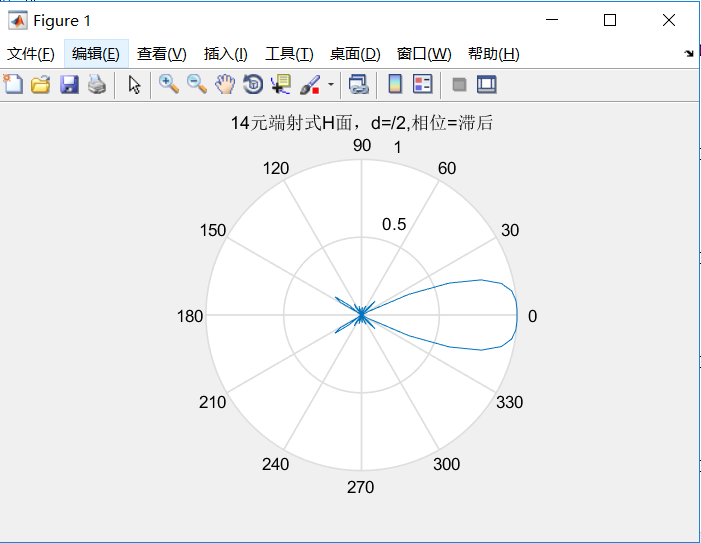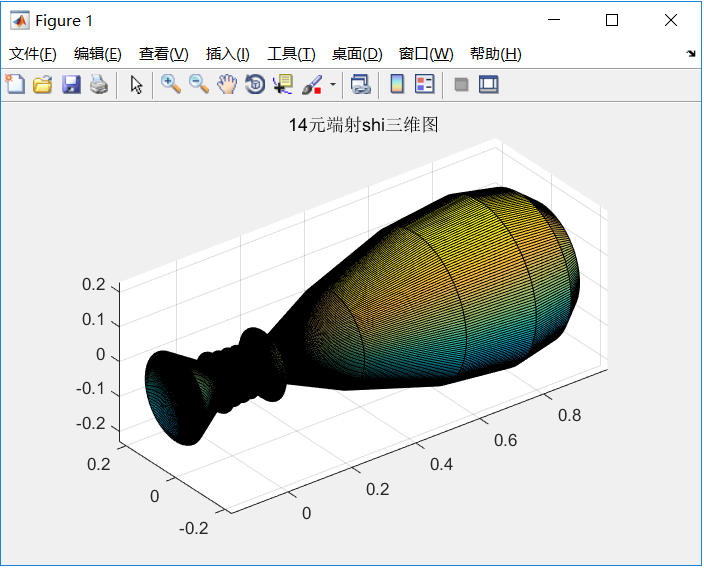a=linspace(0,2*pi)
b=linspace(0,pi)
f=sin((cos(a).*sin(b)-1)*(9/2)*pi)./(sin((cos(a).*sin(b)-1)*pi/2)*9)
polar(a,f.*sin(b))
title('9元端射式H面，d=/2,相位=滞后')
y1=(f.*sin(a))'*cos(b)
z1=(f.*sin(a))'*sin(b)
x1=(f.*cos(a))'*ones(size(b))
surf(x1,y1,z1)
axis equal
title('9元端射式三维图')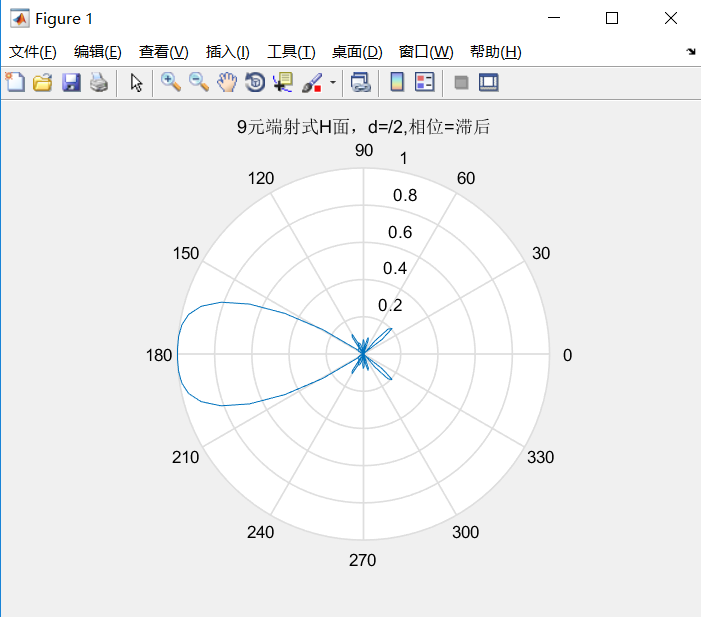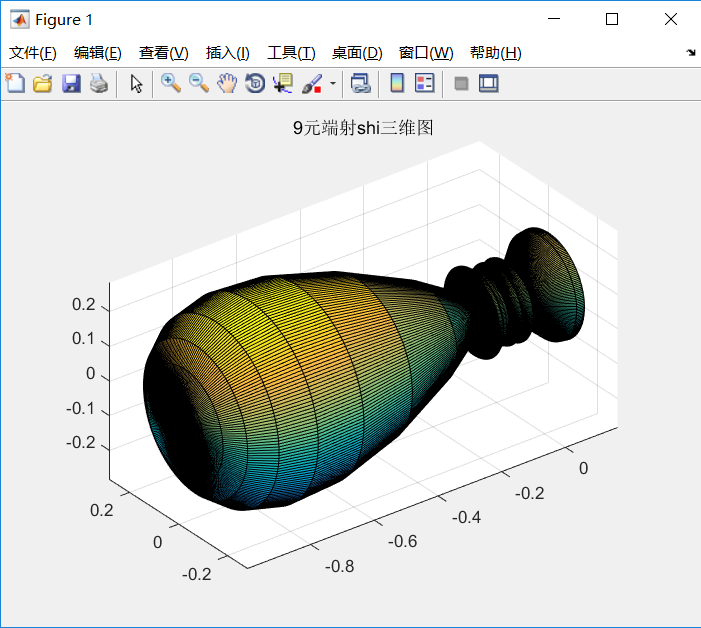a=linspace(0,2*pi)
b=linspace(0,pi)
f=sin((cos(a).sin(b)-1)(6/2)*pi)./(sin((cos(a).*sin(b)-1)*pi/2)*6)
polar(a,f.*sin(b))
title(‘6元端射式H面，d=/2,相位=滞后’)
y1=(f.*sin(a))’*cos(b)
z1=(f.*sin(a))’*sin(b)
x1=(f.*cos(a))‘ones(size(b))
surf(x1,y1,z1)
axis equal
title(‘6元端射式三维图’)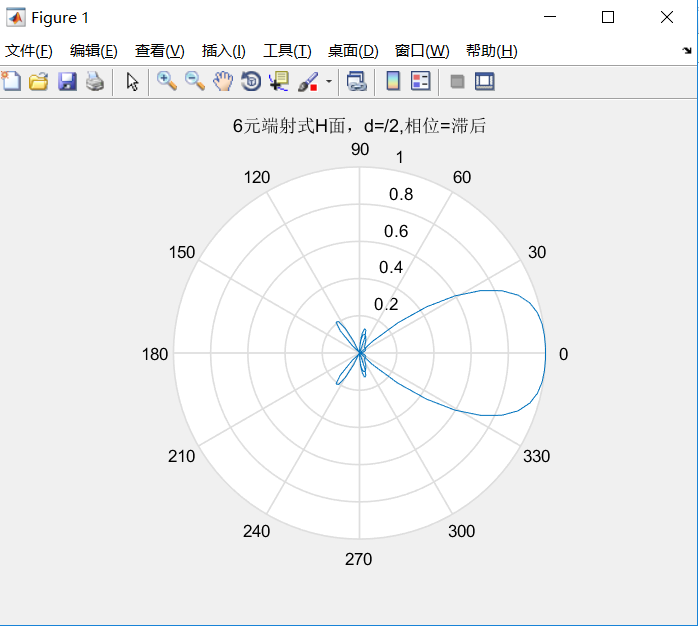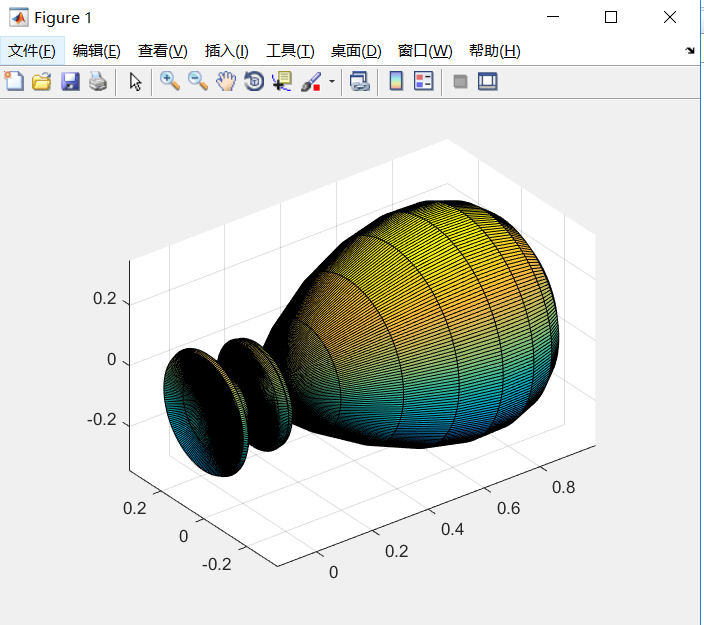a=linspace(0,2pi)
b=linspace(0,pi)
f=sin((cos(a).sin(b)-1)(4/2)*pi)./(sin((cos(a).*sin(b)-1)*pi/2)*4)
polar(a,f.*sin(b))
title(‘4元端射式H面，d=/2,相位=滞后’)
y1=(f.*sin(a))’*cos(b)
z1=(f.*sin(a))’*sin(b)
x1=(f.*cos(a))’*ones(size(b))
surf(x1,y1,z1)
axis equal
title(‘4元端射式三维图’)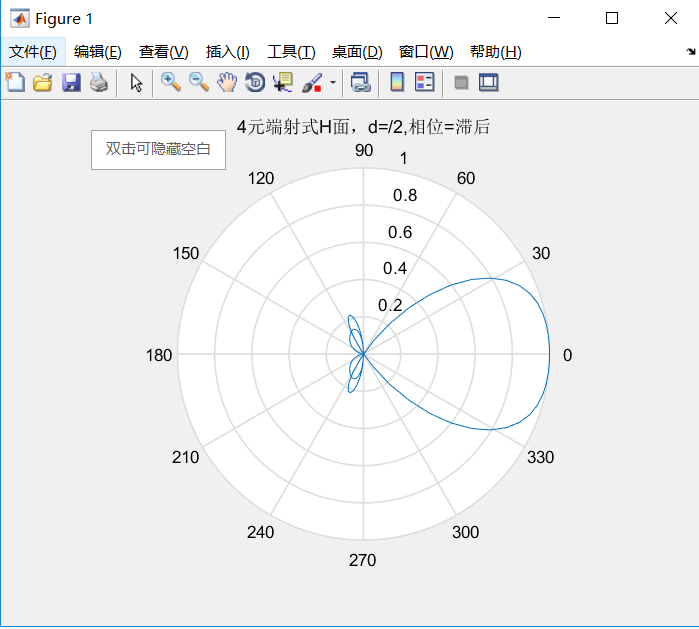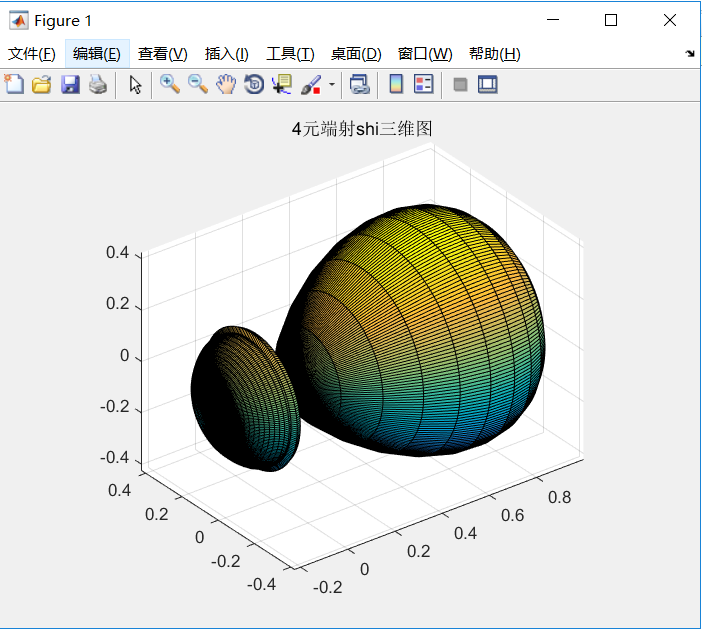从上图可以看出,天线元数目N越大，间距d越大，主瓣就越窄。d也不能任意加大,间距d一般为K/2,在间距一定的情况下，从图3至图8可看出，随着N数目的变化单向端射阵的主瓣宽度也随之变化。天线元数目N超过9后，主瓣宽度的变化不是太明显了。强端射阵不是靠增加N的数目，而是调整天线元之间的相位差D即可。



展开全文• 关于天线方向图的详细介绍，其中详细介绍了方向图的定义和很多有用的图示，读者可以受益良多
• 针对实验室小型天线工程测试的需要，根据天线方向图测试原理，设计开发了天线方向图自动测试系统。该系统以MSP430单片机为核心，由自动控制模块、信号采集存储模块和数据处理显示模块三部分组成。从实验结果来看，该...
• 一段微波画天线方向图的matlab小程序，仅供参考
• 天线方向图又叫辐射方向图（radiation pattern）、远场方向图（far-field pattern）。从方向图上面不能得到天线增益，由方向图得到的是方向系数。天线增益=方向系数 * 天线效率。所以 方向系数 大于 增益 是肯定的...


pattern）、远场方向图（far-field pattern）。从方向图上面不能得到天线增益，由方向图得到的是方向系数。天线增益=方向系数
* 天线效率。所以 方向系数 大于 增益 是肯定的。

天线增益主要是通过方向图的测试而表现出来.这里有很多的种测试方向图的测试系统.也就是暗室.而在暗室的测试出来的结果,也只是一种和理想对称振子比较的的结果.都知道理想对称振子的增益为2.15dB.这样就可以根据测试电平的高低来计算出天线的增益.G=D*N%.而天线的效率一般情况下是没有百分百的，所以G<d
。在计算天线的方向系数D是,通常所采用的就是根据方向图上面表现出来的主瓣的波瓣宽度计算,如半功率波瓣宽度,也就是电平下降3dB是的波瓣宽度.

天线增益：

天线增益是指：在输入功率相等的条件下，实际天线与理想的辐射单元在空间同一点处所产生的信号的功率密度之比。它定量地描述一个天线把输入功率集中辐射的程度。增益显然与天线方向图有密切的关系，方向图主瓣越窄，副瓣越小，增益越高。天线增益是用来衡量天线朝一个特定方向收发信号的能力，它是选择基站天线最重要的参数之一。一般来说，增益的提高主要依靠减小垂直面向辐射的波瓣宽度，而在水平面上保持全向的辐射性能。天线增益对移动通信系统的运行质量极为重要，因为它决定蜂窝边缘的信号电平。增加增益就可以在一确定方向上增大网络的覆盖范围，或者在确定范围内增大增益余量。任何蜂窝系统都是一个双向过程，增加天线的增益能同时减少双向系统增益预算余量。另外，表示天线增益的参数有dBd和dBi。DBi是相对于点源天线的增益，在各方向的辐射是均匀的；dBd相对于对称阵子天线的增益dBi=dBd+2.15。相同的条件下，增益越高，电波传播的距离越远。一般地，GSM定向基站的天线增益为18dBi，全向的为11dBi。

天线方向图的特征参数：

为了方便对各种天线的方向图特性进行比较，就需要规定一些特性参数。主要包括：主瓣宽度，旁瓣电平，前后比，方向系数等1.主瓣宽度：是衡量天线的最大辐射区域的尖锐程度的物理量。通常取天线方向图主瓣两个半功率点之间的宽度。2.旁瓣电平：是指离主瓣最近且电平最高的第一旁瓣的电平，一般以分贝表示。3.前后比：是指最大辐射方向（前向）电平与其相反方向（后向）电平之比，通常以分贝为单位。4.方向系数：在离天线某一距离处，天线在最大辐射方向上的辐射功率流密度与相同辐射功率的理想无方向性天线在同一距离处的辐射功率流密度之比。

天线的方向图作图：

天线的方向图是表征天线辐射特性(场强振幅、相位、极化)与空间角度关系的图形。完整的方向图是一个三维的空间图形，如图3.1所示。它是以天线相位中心为球心（坐标原点），在半径r足够大的球面上，逐点测定其辐射特性绘制而成。测量场强振幅，就得到场强方向图；测量功率，就得到功率方向图；测量极化，就得到极化方向图；测量相位，就得到相位方向图。若不另加说明，本书说述方向图均指场强振幅方向图。三维空间方向图的测绘十分麻烦，实际工作中，一般只需测得水平面和垂直面（即XY平面和XZ平面）的方向图就行了。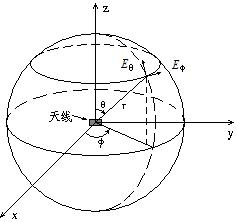图1 测量方向图的坐标

天线方向图可以用极坐标绘制，也可以用直角坐标绘制。极坐标方向图的特点是直观、简单，从方向图可以直接看出天线辐射场强的空间分布特性。但当天线方向图的主瓣窄而副瓣电平低时，直角坐标绘制法显示出更大的优点。因为表示角度的横坐标和表示辐射强度的纵坐标均可任意选取，例如即使不到1°的主瓣宽度也能清晰地表示出来，而极坐标却无法绘制。图2所示为同一天线方向图的两种坐标表示法。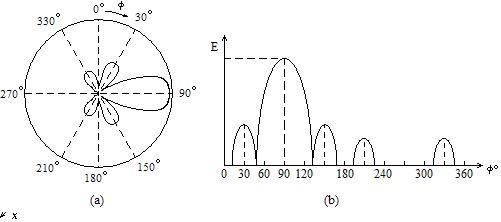图2方向图的表示法 (a)极坐标 (b)直角坐标

一般绘制方向图时都是经过归一化的，即径向长度(极坐标)或纵坐标值(直角坐标)是以相对场强E（θ，φ）/Emax，这里E（θ，φ）是任一方向的场强值，Emax是最大辐射方向的场强值。因此，归一化最大值是1。对于极低副瓣电平天线的方向图，大多采用分贝值表示，归一化最大值取为零分贝。图3所示为直角坐标中用归一化场强和分贝值表示的同一天线方向图。
图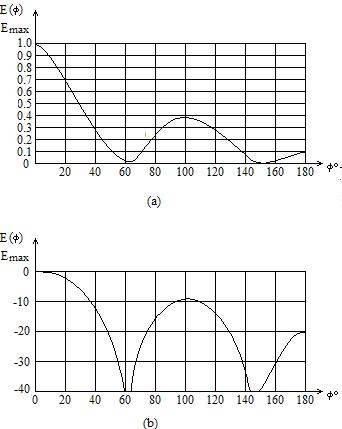图3 归一化方向图


展开全文• 天线的方向性是指电磁场辐射在空间的分布...讨论了阵列天线方向图中主射方向和主瓣宽度随各参数变化的特点,借助MATLAB绘制出天线方向性因子的二维和三维方向图,展示天线辐射场在空间的分布规律,表现辐射方向图的特点。
• 由于采用普通粒子群算法存在着易于早熟和局部寻优能力不足等缺点，为此本文提出一种基于量子位概率幅编码QPSO算法的阵列天线方向图综合技术，即在方向图综合中，QPSO算法采用量子位对粒子当前位置进行编码，用量子...
• 基站天线方向图测试系统的开发，朱菊香，周建良，从天线问世到现在，方向图测量一直成为人们关注的重点课题之一，而今随着现代分析技术和设计的发展，方向图测量变成越来越不能缺
• 行业分类-物理装置-一种天线方向图主瓣可控的天线阵列设计方法.zip
• 行业分类-电子电器-一种天线方向图主瓣可控的天线阵列设计方法.zip
• 基于LCMV算法的天线方向图零陷，王俊勇，，本文讲述在LCMV波束形成中，增加最优权目标拟合函数，得到的方向图能保证期望信号方向增益最大，干扰方向形成零点并且副瓣形状、�
• 与需要用于操纵阵列波束的万向架的反射器天线相比，ESAS在空间中电子地扫描阵列波束而没有阵列的物理移动(参见1.1)。与反射器的毫秒相比，用ESA扫描光束可以在微秒量级上执行。这使得除了灵活性之外，还能够增加......